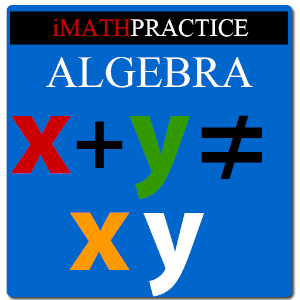# Master Algebra Lite - Lumos Educational App Store3.71
Price -
\$

#### DESCRIPTION:

This is for students in High School/College learning algebra. If you are a beginner in algebra you might be thinking X+Y=XY, Is not it? But itâs not. The beauty of algebra is, it deals with variables, expressions & equations. You will come to know various formulas. For example if you know (a+b)^3= a^3+b^3+3a^2b+3ab^2. You can calculate any number to the powers 2,3,4â¦in a fraction of seconds. In the above equation a ,b are variables. So you can calculate (1.034)^3 also using that formula. Just feed a=1& b=.034 IMathPractice Algebra's 3 steps method of teaching has sections like T

#### OVERVIEW:

Master Algebra Lite is a free educational mobile app By ongolearn.com.It helps students in grades HS practice the following standards HSN.RN.A.1,HSN.RN.A.2,HSN.RN.B.3.

This page not only allows students and teachers download Master Algebra Lite but also find engaging Sample Questions, Videos, Pins, Worksheets, Books related to the following topics.

1. HSN.RN.A.1 : Explain how the definition of the meaning of rational exponents follows from extending the properties of integer exponents to those values, allowing for a notation for radicals in terms of rational exponents. For example, we define 51/3 to be the cube root of 5 because we want (51/3)3 = 5(1/3)3 to hold, so (51/3)3 must equal 5.

2. HSN.RN.A.2 : Rewrite expressions involving radicals and rational exponents using the properties of exponents..

3. HSN.RN.B.3 : Explain why the sum or product of two rational numbers is rational; that the sum of a rational number and an irrational number is irrational; and that the product of a nonzero rational number and an irrational number is irrational.

HS

HSN.RN.A.1
HSN.RN.A.2
HSN.RN.B.3

#### ADDITIONAL INFORMATION:

Developer: ongolearn.com

Software Version: 1.3

Category: Education

### RELATED APPS